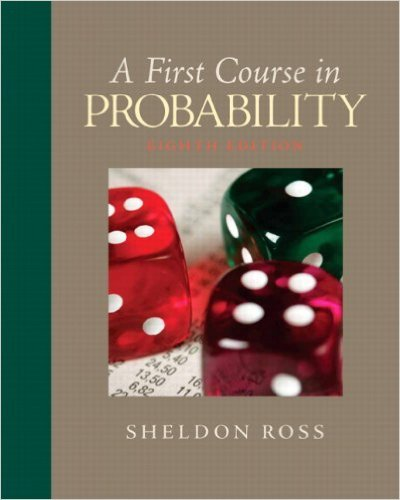# A pair of fair dice is rolled. What is the probabilitythat## Problem 23 Chapter 2

First Course in Probability | 8th Edition

• 2901 Step-by-step solutions solved by professors and subject experts
• Get 24/7 help from StudySoup virtual teaching assistantsFirst Course in Probability | 8th Edition

4 5 0 259 Reviews
12
0
Problem 23

A pair of fair dice is rolled. What is the probabilitythat the second die lands on a higher value thandoes the first?

Step-by-Step Solution:
Step 1 of 3

Review of Notes 4: i = 1, 2 ….. n j = 1, 2 ….r i ̅ ∑ ∑ ̅ ∑ ∑ ∑ ∑ ( ̅) => Overall Variation (SST total sum of squares) ∑ ∑ ( ) = {( ̅ ) ̅ ̅ } = ∑ ∑ ( ̅ ) ∑ ∑...

Step 2 of 3

Step 3 of 3

##### ISBN: 9780136033134

Unlock Textbook Solution

A pair of fair dice is rolled. What is the probabilitythat

×
Get Full Access to First Course In Probability - 8 Edition - Chapter 2 - Problem 23

Get Full Access to First Course In Probability - 8 Edition - Chapter 2 - Problem 23

I don't want to reset my password

Need help? Contact support

Need an Account? Is not associated with an account
We're here to help